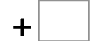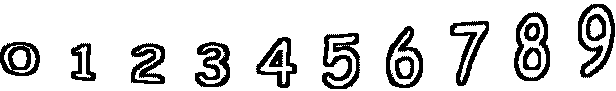0= 5   9= 190= 4   3= 6   9= 13   1= 8 9= 17   7= 11   1= 3   8= 12